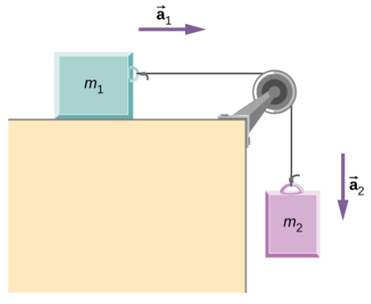# Physicsthe pulley system problem

#### Cbarker1

##### Active member
Two blocks are connected by a massless rope as shown below. The mass of the block on the table is 5.4 kg and the hanging mass is 2.1 kg. The table and the pulley are frictionless.I need to find acceleration, the tension of the rope, and the speed when mass 2 hits the floor when it starts from rest and is initially located 1.3 m from the floor.
I need some help with the setup.

Last edited:

#### MarkFL

Staff member
Let's look at the forces on each object in turn. For $m_1$, we have:

$$\displaystyle \sum F_x=T=m_1a$$

And for $m_2$, we have:

$$\displaystyle \sum F_y=m_2g-T=m_2a$$

Now we have two equations in two unknowns...can you proceed?

To answer the second part of the question, I would use the kinematic equation:

$$\displaystyle \Delta y=\frac{v_f^2-v_i^2}{2a}$$

Solve that for $v_f$, and then use the given values (and the acceleration $a$ you found in the first part) to get your answer.#### MarkFL

Staff member
As a follow-up, we have when substituting for $T$ from the first equation into the second:

$$\displaystyle m_2g-m_1a=m_2a$$

Solving for $a$, we obtain:

$$\displaystyle a=\frac{m_2g}{m_1+m_2}$$

And so:

$$\displaystyle T=\frac{m_1m_2g}{m_1+m_2}$$

And finally:

$$\displaystyle v_f=\sqrt{\frac{2m_2g\Delta y}{m_1+m_2} + v_i^2}$$